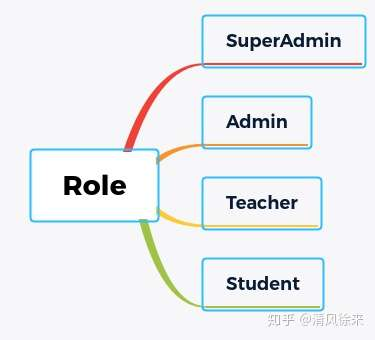2020-12-28 23:50:49 624

简介

1.知识预览

2. click 注册命令，初始化项目基础数据

2.轮子

bbs/extensions.py

db = SQLAlchemy()

3.项目配置

bbs/setting.py

import os

basedir = os.path.abspath(os.path.dirname(os.path.dirname(__file__)))

class BaseConfig(object):
SQLALCHEMY_TRACK_MODIFICATIONS = False

DATABASE_USER = os.getenv('DATABASE_USER')
DATABASE_PWD = os.getenv('DATABASE_PWD')
DATABASE_HOST = os.getenv('DATABASE_HOST')
DATABASE_PORT = os.getenv('DATABASE_PORT')

class DevelopmentConfig(BaseConfig):
SQLALCHEMY_DATABASE_URI = 'mysql+pymysql://{}:{}@{}/bbs?charset=utf8mb4'.format(BaseConfig.DATABASE_USER,
BaseConfig.DATABASE_PWD,
BaseConfig.DATABASE_HOST)
# REDIS_URL = "redis://localhost"
REDIS_URL = "redis://localhost:6379"

• 使用export命令(Linux)
export DATABASE_USER=root
export DATABASE_PWD=123456
export DATABASE_HOST=127.0.0.1
export DATABASE_PORT=3306

• .env文件
可以将这些参数保存到.env文件中，然后通过python-dotenv库自动加载.env文件中的内容到环境变量。在university-bbs目录下新建.env文件，并嵌入下面的代码。
DATABASE_USER=weijiang
DATABASE_PWD=1994124
DATABASE_HOST=127.0.0.1
DATABASE_PORT=3306

4.表的创建

bbs目录下新建一个models.py模块，该模块主要是用来定义我们的数据库表模型用的，嵌入以下代码

bbs/models.py

class User(db.Model, UserMixin):
__tablename__ = 't_user'

id = db.Column(db.INTEGER, primary_key=True, nullable=False, index=True, autoincrement=True)
username = db.Column(db.String(40), nullable=False, index=True, unique=True, comment='user name')
nickname = db.Column(db.String(40), nullable=False, unique=True, comment='user nick name')
email = db.Column(db.String(128), unique=True, nullable=False, comment='user register email')
slogan = db.Column(db.String(40), default='')
website = db.Column(db.String(128), default='', comment="user's website")
location = db.Column(db.String(128), default='', comment='user location')
avatar = db.Column(db.String(100), nullable=False, comment='user avatar')
avatar_raw = db.Column(db.String(100), comment='use avatar raw file')
create_time = db.Column(db.DATETIME, default=datetime.datetime.now)

status_id = db.Column(db.INTEGER, db.ForeignKey('t_status.id'))
college_id = db.Column(db.INTEGER, db.ForeignKey('t_college.id'))
role_id = db.Column(db.INTEGER, db.ForeignKey('t_role.id'), default=3, comment='user role id default is 3 '

college = db.relationship('College', back_populates='user')
role = db.relationship('Role', back_populates='user')
status = db.relationship('Status', back_populates='user')                                                                                      'that is student role')

class College(db.Model):
__tablename__ = 't_college'

id = db.Column(db.INTEGER, primary_key=True, nullable=False, autoincrement=True, index=True)
name = db.Column(db.String(100), nullable=False)
create_time = db.Column(db.DATETIME, default=datetime.datetime.now)

class Role(db.Model):
__tablename__ = 't_role'

id = db.Column(db.INTEGER, primary_key=True, autoincrement=True, index=True)
name = db.Column(db.String(40), nullable=False)
permission_id = db.Column(db.INTEGER, db.ForeignKey('t_permission.id'), nullable=False)

permission = db.relationship('Permission', back_populates='role')

class Status(db.Model):
__tablename__ = 't_status'

id = db.Column(db.INTEGER, primary_key=True, autoincrement=True, index=True)
name = db.Column(db.String(40), nullable=False)

class Permission(db.Model):
__tablename__ = 't_permission'

id = db.Column(db.INTEGER, primary_key=True, autoincrement=True, index=True)
name = db.Column(db.String(40), nullable=False)user = User.query.filter_by(id=1).first()
print(user.role.name)
role = Role.query.filter_by(id=1).first()
print(role.user)

5.初始化基础数据

from bbs.models import *
from bbs.extensions import db
def create_app(config_name=None):
# 省略之前的代码
app.config.from_object(DevelopmentConfig)
register_extensions(app)
return app

db.init_app(app)

def create_app(config_name=None):
# 省略已有代码
register_cmd(app)
return app

@app.cli.command()
click.confirm('这个操作会清空整个数据库,要继续吗?', abort=True)
db.drop_all()
click.echo('清空数据库完成!')
db.create_all()
init_status()
click.echo('初始化状态表完成!')
init_colleges()
click.echo('初始化学院表完成!')
init_permission()
click.echo('初始化权限表完成!')
init_role()
click.echo('初始化角色表完成!')
db.session.commit()
click.echo('数据库初始化完成!')

def init_status():
s1 = Status(name='正常')
s2 = Status(name='禁用')
db.session.commit()

def init_colleges():
colleges = ['计算机科学与技术学院', '信息与通信工程学院', '法学院', '外国语学院', '体育学院', '生命科学学院', '文学院']
for college in colleges:
c = College(name=college)
db.session.commit()

def init_permission():
permissions = ['ALL', 'SOME', 'LITTLE']
for per in permissions:
p = Permission(name=per)
db.session.commit()

def init_role():
roles = ['超级管理员', '老师', '学生']
r1 = Role(name=roles, permission_id=1)
r2 = Role(name=roles, permission_id=2)
r3 = Role(name=roles, permission_id=3)
db.session.commit()